PGA Odds
+240
2.4 to 1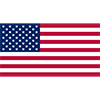Justin Thomas
17.4% implied probability

+550
5.5 to 1Lanto Griffin
9.1% implied probability

+600
6 to 1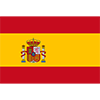Jon Rahm
8.5% implied probability

+700
7 to 1Bubba Watson
7.4% implied probability

+750
7.5 to 1Tony Finau
7.0% implied probability

+1100
11 to 1Patrick Cantlay
4.9% implied probability

+1200
12 to 1Scottie Scheffler
4.6% implied probability

+1400
14 to 1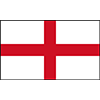Tyrrell Hatton
3.9% implied probability

+1400
14 to 1Patrick Reed
3.9% implied probability

+2000
20 to 1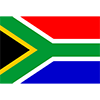Dylan Frittelli
2.8% implied probability

+2000
20 to 1Harris English
2.8% implied probability

+2200
22 to 1Webb Simpson
2.6% implied probability

+2500
25 to 1Brian Harman
2.3% implied probability

+2800
28 to 1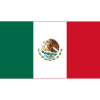Abraham Ancer
2.0% implied probability

+3300
33 to 1Ryan Palmer
1.7% implied probability

+3500
35 to 1Matthew Fitzpatrick
1.6% implied probability

+4000
40 to 1Justin Rose
1.4% implied probability

+5000
50 to 1Kevin Kisner
1.2% implied probability

+5000
50 to 1Jason Kokrak
1.2% implied probability

+5000
50 to 1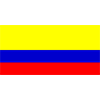Sebastian Munoz
1.2% implied probability

+5000
50 to 1Collin Morikawa
1.2% implied probability

+5000
50 to 1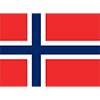Viktor Hovland
1.2% implied probability

+8000
80 to 1Kevin Na
0.7% implied probability

+8000
80 to 1Cameron Champ
0.7% implied probability

+8000
80 to 1Joaquin Niemann
0.7% implied probability